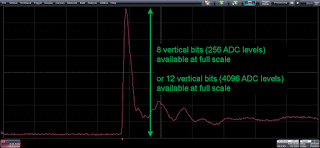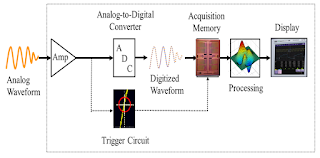## You need to test, we're here to help.

You need to test, we're here to help.

## 30 September 2016

### Dynamic Range, Signal Integrity, and ESD PulsesFigure 1: Use your oscilloscope's full vertical range to take full advantage of the ADC's resolution
Having considered the impact of sampling rate on ESD pulse measurements, let's now turn our attention to dynamic range and signal integrity. No matter what oscilloscope you use, it's important to make the most of the instrument's full vertical range to achieve maximum accuracy.

Figure 1 provides an example of how a pulsed waveform should appear on an oscilloscope's display. When the pulse's full amplitude fills the screen from top to bottom in this fashion, you're getting the most of the available 256 quantization values when using an 8-bit oscilloscope, or the 4096 quantization levels you get from a 12-bit oscilloscope such as Teledyne LeCroy's HDO6000 and HDO8000 series.Figure 2: Using only half of the display's vertical range will give you only half of the ADC's vertical quantization range
Use just half of the vertical scale and your measurements will suffer (Figure 2). How so? In the case of your 8-bit oscilloscope, you'll have reduced its resolution to 7 bits (28/2 = 27). If you're lucky enough to have a 12-bit instrument, using half of the vertical scale will transform it into an 11-bit oscilloscope with 2048 quantization levels, still far better than the 8-bit oscilloscope at full scale but not what it could be.

With only a quarter of the vertical scale in use, the resolution scenario is even more dire. On an 8-bit oscilloscope, you'll now have just 64 quantization levels, or 1024 levels on a 12-bit instrument.

All of this boils down to the fact that what we see on our display isn't just about the visualization of the signal, but rather it reflects what the ADC sees. The key, again, is that no matter what oscilloscope we're using, we want to maximize the display usage so as to not only see the signal better, but to obtain the most accurate characterizations.Figure 3: A simplified block diagram ofa digital oscilloscope
For a better understanding of why maximizing waveforms in the display grid improves measurement accuracy, let's look at a simplified block diagram of an oscilloscope (Figure 3). The input amplifier passes the incoming analog waveform to the ADC. If we use only some fraction of the display, then the ADC uses only that fraction of its range in digitizing the signal. Thus, all measurements are performed at a compromised resolution.

It's important, then, to set the sampling rate to get enough sample points on the rising edge of an ESD pulse. It's also critical to select the volts/div setting that maximizes the oscilloscope's dynamic range. Getting both of these parameters right makes a big difference in obtaining accurate ESD characterizations.